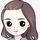cancel
Showing results for
Did you mean:Frequent Visitor

## Average value for last 3 months returns incorrect average

Hi all,I want to calculate the average for the last 3 month and calculate the following formula:

Rolling 3 = CALCULATE(AVERAGE('Master June 2021'[Value (USD)]),DATESINPERIOD('Master June 2021'[Month Year],MAX('Master June 2021'[Month Year]),-3,MONTH))

PB is getting me this result for the 1st set of numbers: 3,268,796.13

But I'm expecting 3,268,821.13 =(3,265,269.82+3,269,615.35+3,271,578.22)/3

Why is it different ?

Thanks
1 ACCEPTED SOLUTIONCommunity Support

Hi @TheBaz_57 ，

In order to give you a suitable solution as soon as possible, could you please share some sample data and field settings for your matrix visual? If any measure or calculated column are referenced, please provide their formulas along with them.Alternatively, you can refer to the following link to try to solve your problem.

Rolling 12 Months Average in DAX

``````CALCULATIONITEM 'PriceToUse'[Moving Average]."Rolling Avg 3M" =
VAR NumOfMonths = 3
VAR LastCurrentDate =
MAX ( 'Date'[Date] )
VAR Period =
DATESINPERIOD ( 'Date'[Date], LastCurrentDate, - NumOfMonths, MONTH )
VAR Result =
CALCULATE (
AVERAGEX (
VALUES ( 'Date'[Calendar Year Month] ),
SELECTEDMEASURE ()
),
Period
)
VAR FirstDateInPeriod = MINX ( Period, 'Date'[Date] )
VAR LastDateWithSales = MAX ( Sales[Order Date] )
RETURN
IF ( FirstDateInPeriod <= LastDateWithSales, Result )``````

Rolling Averages In Power BI

Incorrect total values fix methods:

Why Your Total Is Incorrect In Power BI - The Key DAX Concept To Understand

Dax for Power BI: Fixing Incorrect Measure Totals

Best Regards

Community Support Team _ Rena
If this post helps, then please consider Accept it as the solution to help the other members find it more quickly.Community Support

Hi @TheBaz_57 ，

In order to give you a suitable solution as soon as possible, could you please share some sample data and field settings for your matrix visual? If any measure or calculated column are referenced, please provide their formulas along with them.Alternatively, you can refer to the following link to try to solve your problem.

Rolling 12 Months Average in DAX

``````CALCULATIONITEM 'PriceToUse'[Moving Average]."Rolling Avg 3M" =
VAR NumOfMonths = 3
VAR LastCurrentDate =
MAX ( 'Date'[Date] )
VAR Period =
DATESINPERIOD ( 'Date'[Date], LastCurrentDate, - NumOfMonths, MONTH )
VAR Result =
CALCULATE (
AVERAGEX (
VALUES ( 'Date'[Calendar Year Month] ),
SELECTEDMEASURE ()
),
Period
)
VAR FirstDateInPeriod = MINX ( Period, 'Date'[Date] )
VAR LastDateWithSales = MAX ( Sales[Order Date] )
RETURN
IF ( FirstDateInPeriod <= LastDateWithSales, Result )``````

Rolling Averages In Power BI

Incorrect total values fix methods:

Why Your Total Is Incorrect In Power BI - The Key DAX Concept To Understand

Dax for Power BI: Fixing Incorrect Measure Totals

Best Regards

Community Support Team _ Rena
If this post helps, then please consider Accept it as the solution to help the other members find it more quickly.